# 马尔科夫随机场之图像分割【二】

## 由于经常有读者说运行出错，我又本地校准了下程序，由于版权限制，lena图MATLAB新版已经撤除了，这里改成了cameraman的图

刘伟强等；基于马尔科夫随机场的遥感图像分割和描述；东南大学学报；（29）：11-15，1999

version:2017.1.20 基本理解框架

Markov Random Filed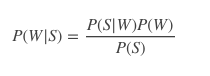• W就是要求的分类标签，称P(W)为标记场w的先验概率（要求它，事先又不知道，那就叫先验概率吧，不知道是不是这样来的）。
• P(S|W)是观察值S的条件概率分布（也称似然函数），看结构为在已知像素点标记w情况下，它是真实的像素点s概率，所以是一个似然函数，表示我的观察像素点和真实的分类情况w像不像的一种程度。
• P(S)是什么？S是观察到的图像，在分割前它就已经定了，我就要分割这幅图像，那它还有概率吗？没有吧，所以P(S)认为是个定值。

Hammersley-Clifford定理可知，要定义一个马尔科夫随机场，由于它与一个吉布斯随机场相对应，如果定义了该吉布斯随机场的能量函数，那么这个马尔科夫随机场也就确定了

MAP：Maximum A Posterior，最大后验概率估计

Ok，既然我们认为每个像素点的分类符合马尔科夫随机模型，而另一些学者证明了这种马尔科夫随机场可以与一个Gibbs随机场等价（这就是Clifford-Hammersley定理，人家证明的，那就这样叫吧）。而Gibbs随机场也有一个概率密度函数，这样我们就用求图像的Gibbs随机场的概率P代替了P(W)。那么Gibbs随机场的概率P怎么表示呢？如下：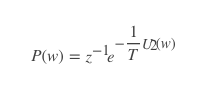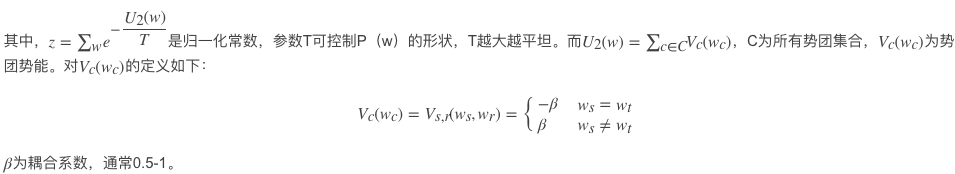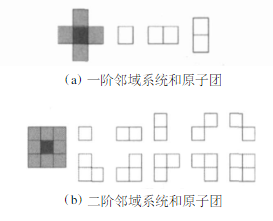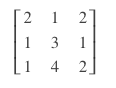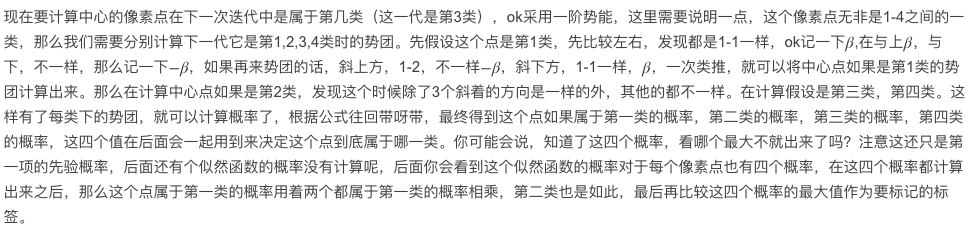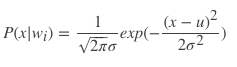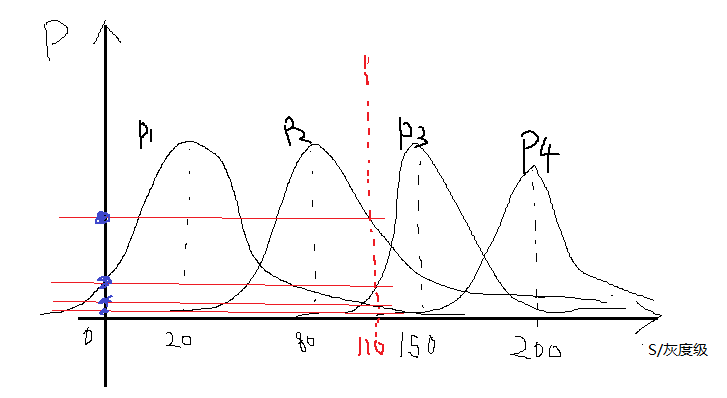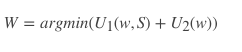clc;clear;close all
cluster_num = 4;%设置分类数
maxiter = 60;%最大迭代次数
%-------------随机初始化标签----------------
label = randi([1,cluster_num],size(img));
%-----------kmeans最为初始化预分割----------
% label = kmeans(img(:),cluster_num);
% label = reshape(label,size(img));
for iter=0:maxiter-1
%-------计算先验概率---------------
%这里采用的是像素点和3*3领域的标签相同
%与否来作为计算概率
%------收集上下左右斜等八个方向的标签--------
label_u = imfilter(label,[0,1,0;0,0,0;0,0,0],'replicate');
label_d = imfilter(label,[0,0,0;0,0,0;0,1,0],'replicate');
label_l = imfilter(label,[0,0,0;1,0,0;0,0,0],'replicate');
label_r = imfilter(label,[0,0,0;0,0,1;0,0,0],'replicate');
label_ul = imfilter(label,[1,0,0;0,0,0;0,0,0],'replicate');
label_ur = imfilter(label,[0,0,1;0,0,0;0,0,0],'replicate');
label_dl = imfilter(label,[0,0,0;0,0,0;1,0,0],'replicate');
label_dr = imfilter(label,[0,0,0;0,0,0;0,0,1],'replicate');
p_c = zeros(4,size(label,1)*size(label,2));
%计算像素点8领域标签相对于每一类的相同个数
for i = 1:cluster_num
label_i = i * ones(size(label));
temp = ~(label_i - label_u) + ~(label_i - label_d) + ...
~(label_i - label_l) + ~(label_i - label_r) + ...
~(label_i - label_ul) + ~(label_i - label_ur) + ...
~(label_i - label_dl) +~(label_i - label_dr);
p_c(i,:) = temp(:)/8;%计算概率
end
p_c((p_c == 0)) = 0.001;%防止出现0
%---------------计算似然函数----------------
mu = zeros(1,4);
sigma = zeros(1,4);
%求出每一类的的高斯参数--均值方差
for i = 1:cluster_num
index = find(label == i);%找到每一类的点
data_c = img(index);
mu(i) = mean(data_c);%均值
sigma(i) = var(data_c);%方差
end
p_sc = zeros(4,size(label,1)*size(label,2));
%     for i = 1:size(img,1)*size(img,2)
%         for j = 1:cluster_num
%             p_sc(j,i) = 1/sqrt(2*pi*sigma(j))*...
%                 exp(-(img(i)-mu(j))^2/2/sigma(j));
%         end
%     end
%------计算每个像素点属于每一类的似然概率--------
%------为了加速运算，将循环改为矩阵一起操作--------
for j = 1:cluster_num
MU = repmat(mu(j),size(img,1)*size(img,2),1);
p_sc(j,:) = 1/sqrt(2*pi*sigma(j))*exp(-(img(:)-MU).^2/2/sigma(j));
end
%找到联合一起的最大概率最为标签，取对数防止值太小
[~,label] = max(log(p_c) + log(p_sc));
%改大小便于显示
label = reshape(label,size(img));
%---------显示----------------
if ~mod(iter,6)
figure;
n=1;
end
subplot(2,3,n);
imshow(label,[])
title(['iter = ',num2str(iter)]);
pause(0.1);
n = n+1;
end

1. 现在假设把图像分两类cluster_num=2，采用随机初始化分类，贴几个之间结果：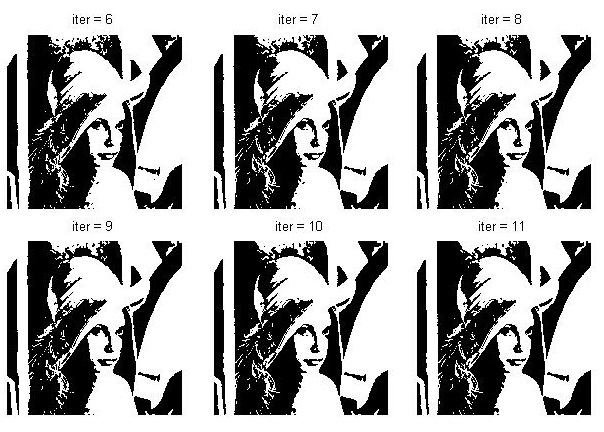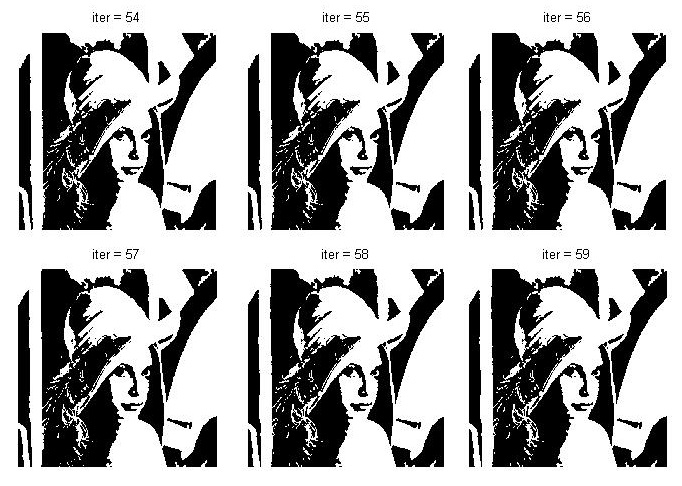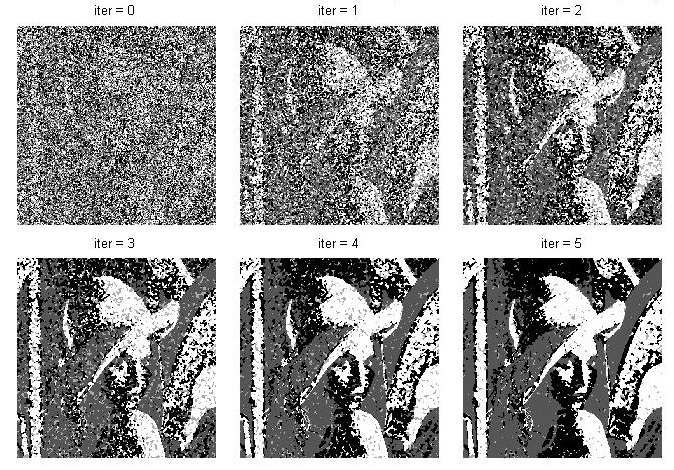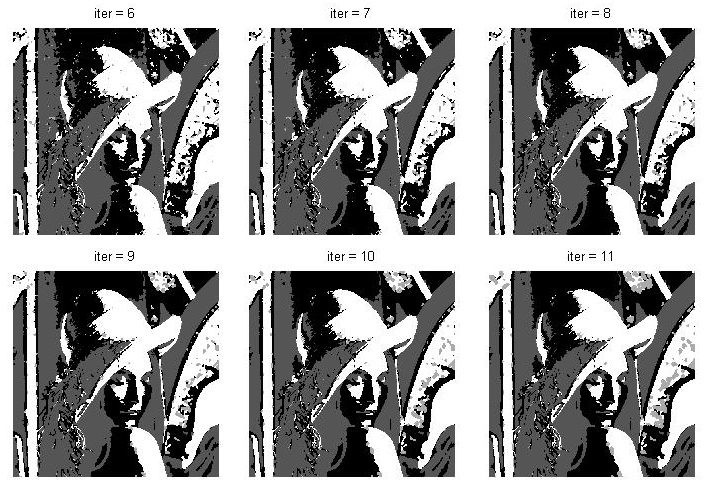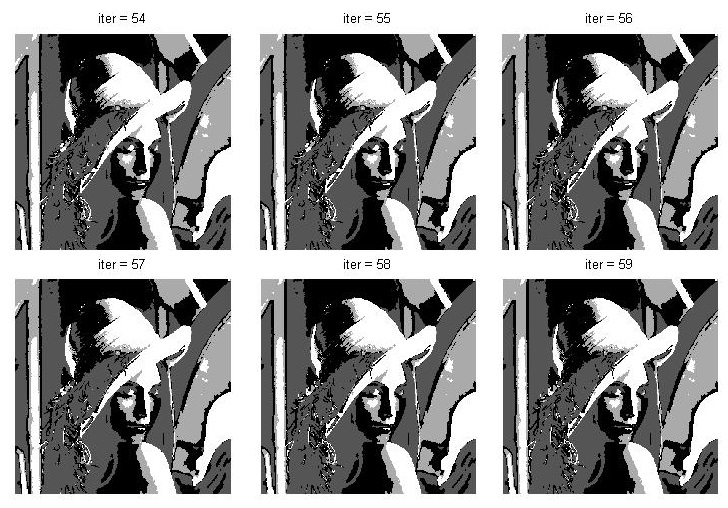05-18100606-061万+
04-19321
11-12495
09-17198
08-094万+
11-101996
11-256133
09-2041
12-022726